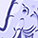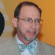cancel
Showing results for
Did you mean:New Contributor

## What is the answer to this school Problem??

We have a problem in chemistry class and we are incapable of agreeing on an answer can someone help, please!

To create Champagne, we ferment raisin jus in a closed bottle to create bubbly wine. the réaction transforms the sugar (C6H12O6) into ethanol (C2H5OH) and into carbon dioxide with the following formula:

C6H12O6 --> 2 C2H5OH + 2 CO2

We ferment 750 mL of raisin jus in a 900 mL bottle until 15% of the initial mass of raisin jus transforms into ethanol. Knowing that the volumic mass of raisin jus is 1,0g/mL and that the one of ethanol is 0.79 g/mL, what will be the pressure of the carbon dioxide in the bottle if the temperature is of 25 C? Suppose that the carbone dioxide does not dissolve in water.

Thank you! Know that we mostly use the formula PV=nRT so that is neededthank you for all helpContributor III

## Re: What is the answer to this school Problem??

Dear Miguel,

First, develop all of the quantities that you will need for a solution. The final solution requested is a molar volume of carbon dioxide in the headspace of a container.

The container is 900mL, of which 750mL is filled with juice, leaving 150mL as the “working space” for the molar ratio and pressure calculations. Using the density of the raisin juice you can obtain its mass. From that you then obtain the 15% that is converted ethanol. If the density of ethanol was given in order to make a better calculation of the remaining liquid volume (and resulting headspace), then you need to adjust the original 750mL at 1g/mL to the volume of a mix containing the given ratio of ethanol to juice with their respective densities.

Using the molecular mass of ethanol you can derive the number of moles produces, and then from your equation you can calculate the number of moles of carbon dioxide produced. Using the revised headspace volume, the given temperature and the assumption of 1atm pressure (air) in the container headspace you can calculate the partial pressure exerted by the carbon dioxide and the total pressure of the headspace after carbon dioxide generation.

In reality of course, a good part of the carbon dioxide is dissolved in the liquid (that’s what MAKES it “Champagne”!). That amount is also both pressure and temperature dependent. Fortunately, it is ignored in this problem.

Always remember that you learn BEST by DOING, not by watching others do it!

Best regards,

Steven